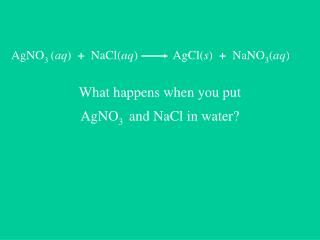DownloadDownload PresentationAgNO 3 ( aq ) + NaCl( aq ) AgCl( s ) + NaNO 3 ( aq )

# AgNO 3 ( aq ) + NaCl( aq ) AgCl( s ) + NaNO 3 ( aq )

Télécharger la présentation## AgNO 3 ( aq ) + NaCl( aq ) AgCl( s ) + NaNO 3 ( aq )

- - - - - - - - - - - - - - - - - - - - - - - - - - - E N D - - - - - - - - - - - - - - - - - - - - - - - - - - -
##### Presentation Transcript

1. AgNO3 (aq) + NaCl(aq) AgCl(s) + NaNO3(aq) What happens when you put AgNO3 and NaCl in water?

2. AgNO3 (aq) + NaCl(aq) AgCl(s) + NaNO3(aq) What really happens when you put AgNO3 and NaCl in water?

3. KEY POINT: NaCl (aq) really means: Na +1(aq) + Cl -1(aq) “dissociated ions” or “ions in solution”

4. What is Dissolving ? When an ionic compound (eg salt) dissolves in water, the compound disassociates. (breaks apart into cations and anions) Ex: Ca(NO3)2(s)Ca2+(aq) + 2NO3-(aq) When a covalent compound (eg sugar) dissolves in water, the molecules simply disperse; they do not disassociate. Molecules of the covalent compounds simply disperse due to attraction with polar water molecules.

5. An Ionic Compound Dissolves: A Covalent Compound Dissolves:

6. KEY POINT: Mg(NO3)2(aq) really means: Mg +2(aq) + (NO3) -1(aq) 2 “dissociated ions” or “ions in solution”

7. KEY POINT: AgCl (s) really means: AgCl (s) Solid Silver (I) Chloride.

8. AgNO3 (aq) + NaCl(aq) AgCl(s) + NaNO3(aq) What happens when you put AgNO3 and NaCl in water?

9. AgNO3 NaCl

10. NO3- Na+ Ag+ Cl-

11. Na+ NO3- Ag+ Cl-

12. Na+ NO3- AgCl

13. Na+ NO3- AgCl(s) precipitate AgCl

14. NO3- Na+ NO3- NO3- Na+ Na+ Na+ NO3- NO3- Na+ Na+ Na+ NO3- NO3- Na+ NO3- Na+ AgCl(s)

15. AgNO3 (aq) + NaCl(aq) AgCl(s) + NaNO3(aq) complete ionic equation

16. AgCl Na+ NO3- Reaction

17. No Reaction Na+ NO3- AgCl

18. These ions do not participate in the reaction. They are called SPECTATOR IONS Na+ NO3- AgCl

19. The net ionic equation is constructed from the complete ionic equation:

20. NO3- and Na+ are not participating in the reaction

21. net ionic equation

22. net ionic equation

23. Complete molecular equation- • describes double replacement reaction • Complete ionic equation- • more accurately shows the reacting species as ions and the products either as ions or a precipitate • Net ionic equation- • focuses only on the ions REACTING • Spectator ions are those ions that do NOT participate in the reaction

24. PREDICTING THE FORMATION OF A PRECIPITATE • Consider the possibility that a precipitate may form. • Must use the solubility table on page 227 to decide. • Possible outcomes • No visible reaction (nvr) • Formation of one ppt. • Formation of two ppt. (rare occurrence)

25. Basic Chemical equation AgNO3(aq) + KCl(aq)AgCl(s) + KNO3(aq) Complete ionic equation shows ions in solution Ag+ (aq)+NO3(aq) + K+ (aq)+Cl-(aq)AgCl(s) + K+ (aq) + NO3-(aq) Net ionic equation shows ions in rxn Ag+ (aq)+Cl-(aq)AgCl(s) (leave out spectator ions) link